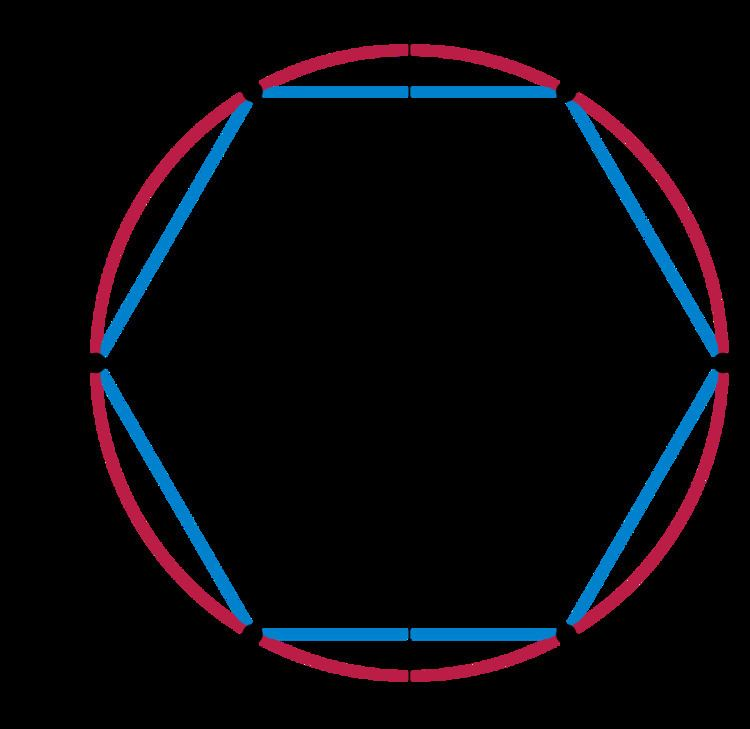# Loop group

Updated onIn mathematics, a loop group is a group of loops in a topological group G with multiplication defined pointwise.

## Definition

In its most general form a loop group is a group of mappings from a manifold M to a topological group G.

More specifically, let M = S1, the circle in the complex plane, and let LG denote the space of continuous maps S1G, i.e.

L G = { γ : S 1 G | γ C ( S 1 , G ) } ,

equipped with the compact-open topology. An element of LG is called a loop in G. Pointwise multiplication of such loops gives LG the structure of a topological group. Parametrize S1 with θ,

γ : θ S 1 γ ( θ ) G ,

and define multiplication in LG by

( γ 1 γ 2 ) ( θ ) γ 1 ( θ ) γ 2 ( θ ) .

Associativity follows from associativity in G. The inverse is given by

γ 1 : γ 1 ( θ ) γ ( θ ) 1 ,

and the identity by

e : θ e G .

The space LG is called the free loop group on G. A loop group is any subgroup of the free loop group LG.

## Examples

An important example of a loop group is the group

Ω G

of based loops on G. It is defined to be the kernel of the evaluation map

e 1 : L G G ,

and hence is a closed normal subgroup of LG. (Here, e1 is the map that sends a loop to its value at 1.) Note that we may embed G into LG as the subgroup of constant loops. Consequently, we arrive at a split exact sequence

1 Ω G L G G 1 .

The space LG splits as a semi-direct product,

L G = Ω G G .

We may also think of ΩG as the loop space on G. From this point of view, ΩG is a H-space with respect to concatenation of loops. On the face of it, this seems to provide ΩG with two very different product maps. However, it can be shown that concatenation and pointwise multiplication are homotopic. Thus, in terms of the homotopy theory of ΩG, these maps are interchangeable.

Loop groups were used to explain the phenomenon of Bäcklund transforms in soliton equations by Chuu-Lian Terng and Karen Uhlenbeck.

## References

Similar Topics
Oil and Water (film)
Chan Yiu Lun
Julia Lashae
Topics